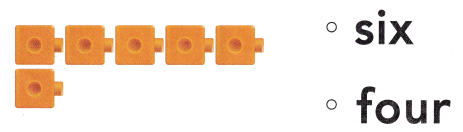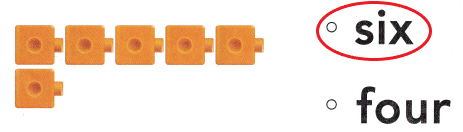# Texas Go Math Kindergarten Lesson 4.2 Answer Key Count and Write 6

Refer to our Texas Go Math Kindergarten Answer Key Pdf to score good marks in the exams. Test yourself by practicing the problems from Texas Go Math Kindergarten Lesson 4.2 Answer Key Count and Write 6.

## Texas Go Math Kindergarten Lesson 4.2 Answer Key Count and Write 6

Explore

DIRECTIONS: Count and tell how many cubes. Trace and write the numbers. Count and tell how many hats. Trace the word.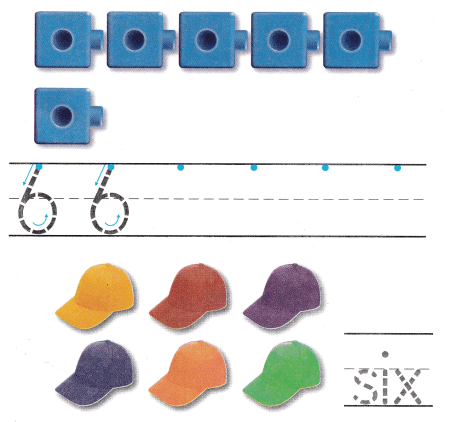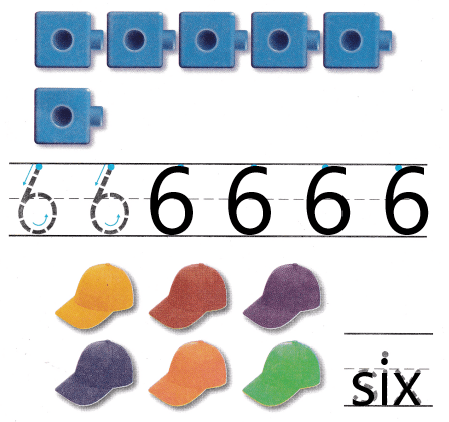Explanation:
I counted the number of cubes.There are 6 cubes.So, i traced the number 6.
I counted the number of hats.There are 6 hats.So, i traced the word six.

Share and Show

DIRECTIONS: 1. Look at the picture. Circle the sets of six objects.

Question 1.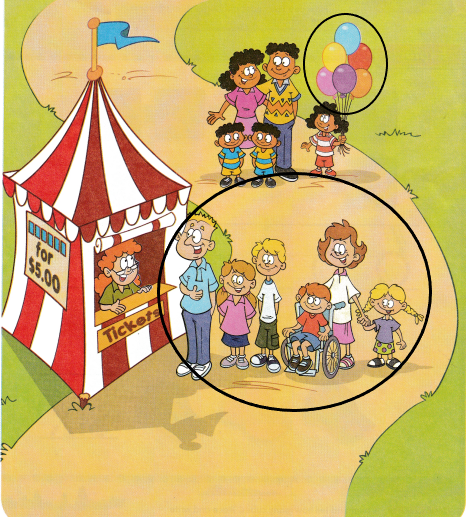Explanation:
I circled the groups that are 6 in number.There are 6 balloons and 6 people in a queue to buy tickets.

DIRECTIONS: 2. Say the number. Practice writing the number. 3-6. Count and tell how many. Write the number.

Question 2.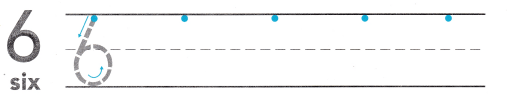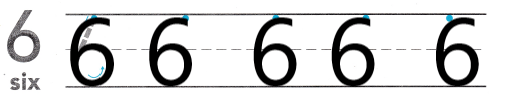Explanation:
I traced and practiced writing the number 6.

Question 3.Explanation:
I counted the number of objects in the image.There are 5 flowers.So, i wrote the number 5.

Question 4.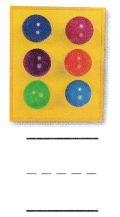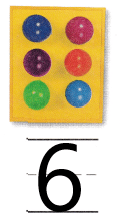Explanation:
I counted the number of objects in the image.There are 6 buttons.So, i wrote the number 6.

Question 5.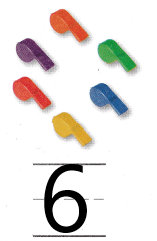Explanation:
I counted the number of objects in the image.There are 6 whistles.So, i wrote the number 6.

Question 6.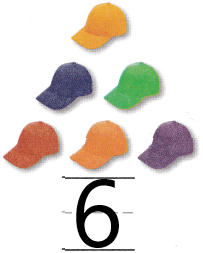Explanation:
I counted the number of objects in the image.There are 6 hats.So, i wrote the number 6.

DIRECTIONS: 7. Marta has a number of whistles that is two less than 6. Count the whistles in each set. Circle the set that shows a number of whistles that is two less than 6. 8. Choose the correct answer. What number does the model show?

Problem Solving

Question 7.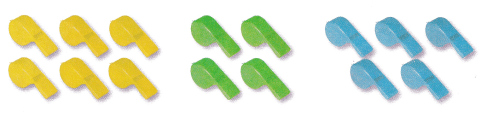Explanation:
I circled the set that shows a number of whistles that is two less than 6.There are 4 green whistles.

Question 8.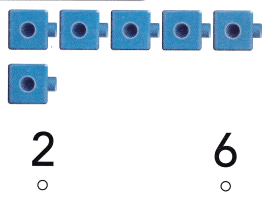###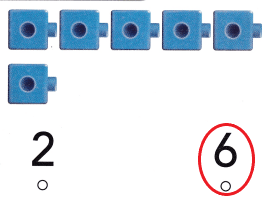Explanation:
I counted the number of cubes.There are 6 cubes.SO, i circled the number 6.

### Texas Go Math Kindergarten Lesson 4.2 Homework and Practice Answer Key

DIRECTIONS: 1-4. Count and tell how many. Write the numbers.

Question 1.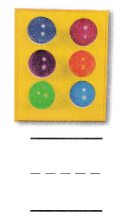Explanation:
I counted the number of objects in the image.There are 6 buttons.So, i wrote the number 6.

Question 2.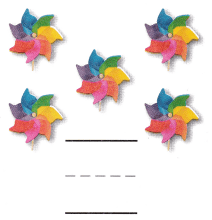Explanation:
I counted the number of objects in the image.There are 5 flowers.So, i wrote the number 5.

Question 3.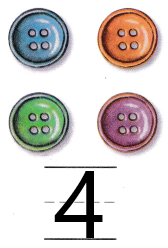Explanation:
I counted the number of objects in the image.There are 4 buttons.So, i wrote the number 4.

Question 4.Explanation:
I counted the number of objects in the image.There are 6 marbles.So, i wrote the number 6.

DIRECTIONS: Choose the correct answer 5-7. Count and tell how many.

Lesson Check

Question 5.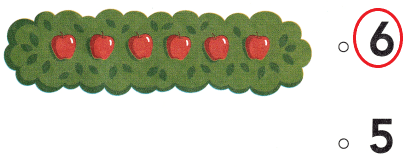Explanation:
There are 6 apples in the image.So, i counted and circled the number 6.

Question 6.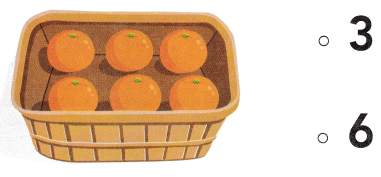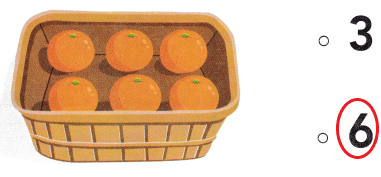Explanation:
There are 6 oranges in the image.So, i counted and circled the number 6.

Question 7.